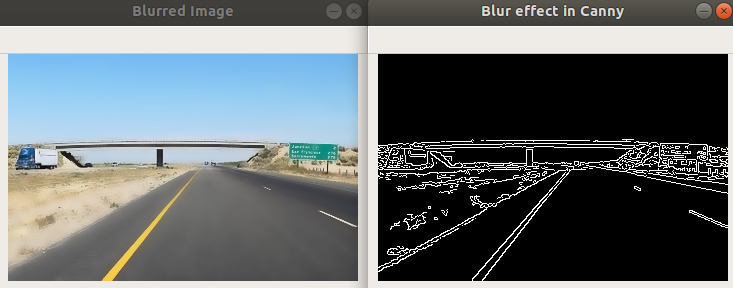# # Blurring and Smoothing Images

## # what is blurring and smoothing images ?

As in normal sense , blurring is the process of making or becoming unclear or less distinct.But this process in computer vision helps to reduce high frequency content (eg: noise, edges) from the image

## # why it is done ?

Often blurring or smoothing is combined with edge detection . It is useful for removing noises. It actually removes high frequency content (eg: noise, edges) from the image. So edges are blurred a little bit in this operation. (Well, there are blurring techniques which doesn't blur the edges too).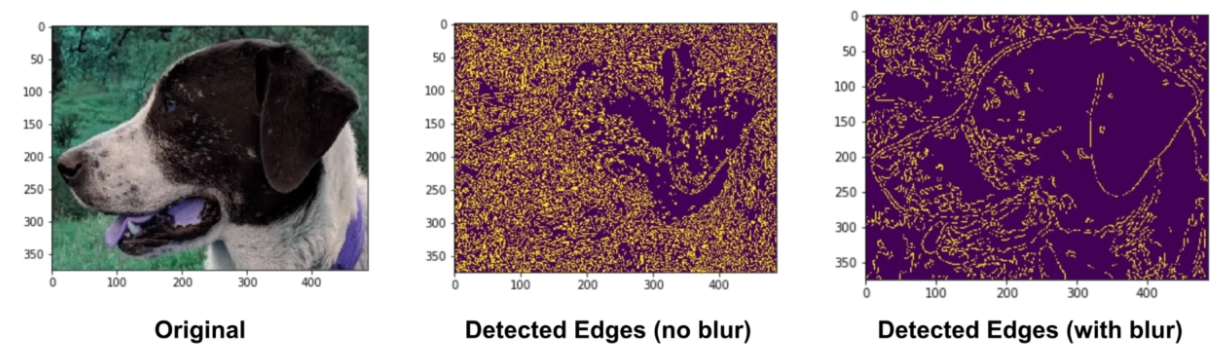## # Types

All image blurring is achieved by convolving the image with a low-pass filter kernel Image Kernels Explained Visually .

OpenCV provides mainly four types of blurring techniques.

1. Averaging:

It simply takes the average of all the pixels under kernel area Image Kernels Explained Visually and replace the central element. This is done by the function `cv2.blur()` or `cv2.boxFilter()`.

``````blur = cv2.blur(img,(5,5)) # (5,5) is kernel size
``````
1

### # RESULT

• (5,5) Kernel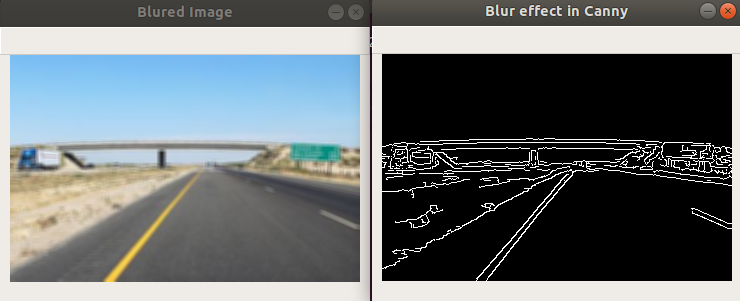• (7,7) Kernel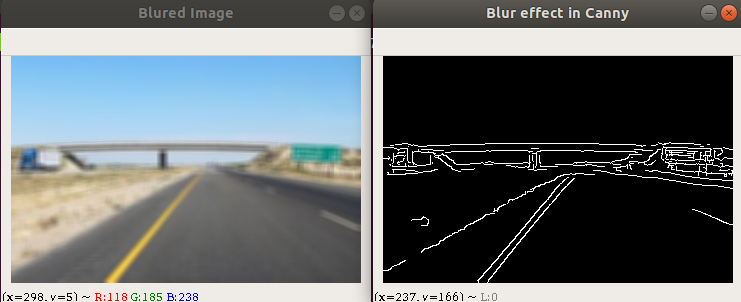1. Gaussian Blurring

In this, instead of box filter, Gaussian kernel is used. It is done with the function, `cv2.GaussianBlur()`. We should specify the width and height of kernel which should be positive and odd. Gaussian blurring is highly effective in removing Gaussian noise from the image.

Gaussian noise is statistical noise having a probability density function (PDF) equal to that of the normal distribution, which is also known as the Gaussian distribution

``````blur = cv2.GaussianBlur(img,(5,5),0)
``````
1

### # RESULT

• (5,5) Kernel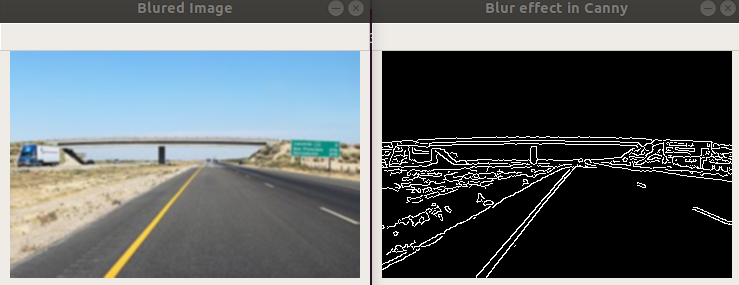• (7,7) Kernel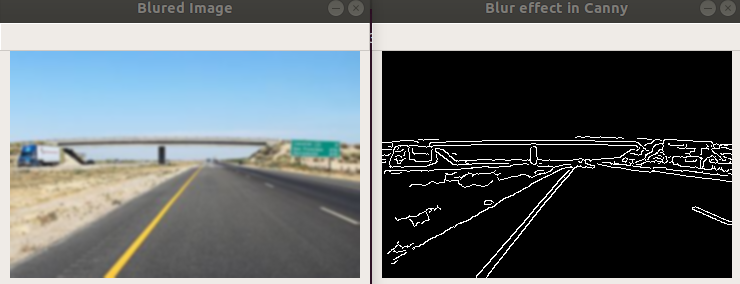1. Median Blurring

Here, the function `cv2.medianBlur()` takes median of all the pixels under kernel area and central element is replaced with this median value.

This is highly effective against salt-and-pepper noise in the images.

Interesting thing is that, in the above filters, central element is a newly calculated value which may be a pixel value in the image or a new value. But in median blurring, central element is always replaced by some pixel value in the image. It reduces the noise effectively. Its kernel size should be a positive odd integer.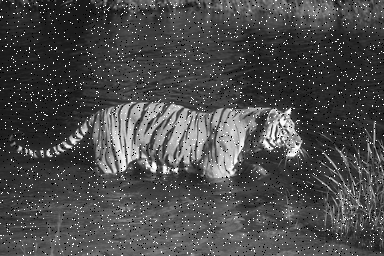salt-and-pepper noise is a form of noise sometimes seen on images. It is also known as impulse noise. This noise can be caused by sharp and sudden disturbances in the image signal.

``````median = cv2.medianBlur(img,5) #5 =kernel size
``````
1

### # RESULT

• (5,5) Kernel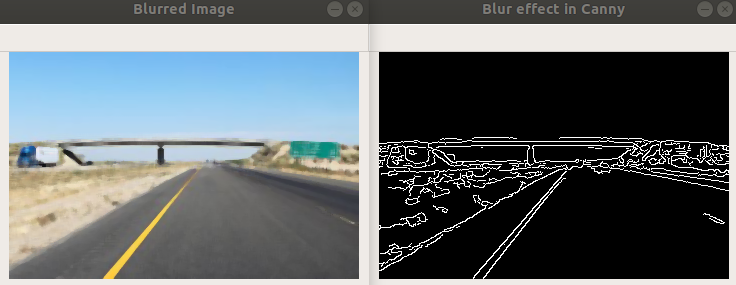• (7,7) Kernel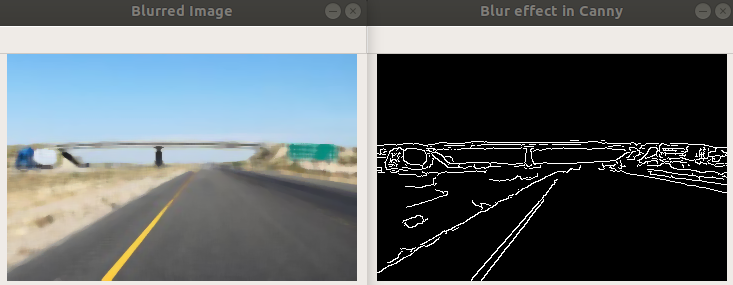1. Bilateral Filtering

`cv2.bilateralFilter()` is highly effective in noise removal while keeping edges sharp. But the operation is slower compared to other filters.

A bilateral filter is non-linear, edge-preserving and noise-reducing smoothing filter. The intensity value at each pixel in an image is replaced by a weighted average of intensity values from nearby pixels. This weight can be based on a Gaussian distribution.

``````#int d =9 ,double sigmaColor = 75,double sigmaSpace 75
# d – Diameter of each pixel neighborhood that is used during filtering. If it is non-positive, it is computed from sigmaSpace .
# sigmaColor – Filter sigma in the color space. A larger value of the parameter means that farther colors within the pixel neighborhood (see sigmaSpace ) will be mixed together, resulting in larger areas of semi-equal color.
# sigmaSpace – Filter sigma in the coordinate space. A larger value of the parameter means that farther pixels will influence each other as long as their colors are close enough (see sigmaColor ). When d>0 , it specifies the neighborhood size regardless of sigmaSpace . Otherwise, d is proportional to sigmaSpace .
blur = cv2.bilateralFilter(img,9,75,75)
``````
1
2
3
4
5

### # RESULT

• d= 20 ,250 ,250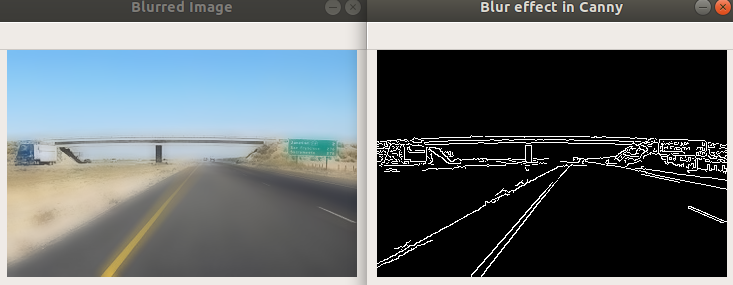• d = 10 ,125 , 125